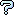All about flooble | fun stuff | Get a free chatterbox | Free JavaScript | Avatarsperplexus dot infoAngle Bisectors (Posted on 2008-02-11)In triangle ABC, angle C has a measure of 60 degrees. Point D lies on side AC so that BD bisects angle B and BD = 1. Similarly, point E lies on side BC so that AE bisects angle A and AE = 2.

Find the area of triangle ABC.

 Submitted by Dennis Rating: 3.0000 (2 votes) Solution: (Hide) Let x = the measure of angle CAE, y = the measure of angle CBD, 120 degrees - x = the measure of angle CEA, and 120 degrees - y = the measure of angle CDB. AC/sin(120-x) = 2/sin(60) --> AC = (2/sqrt(3))(sqrt(3)cosx+sinx) BC/sin(120-y) = 1/sin(60) --> BC = (1/sqrt(3))(sqrt(3)cosy+siny) Now 2x+2y=120 degrees --> y=60-x --> cosy=.5cosx+(sqrt(3)/2)sinx and siny=(sqrt(3)/2)cosx-.5sinx So BC = (1/sqrt(3))(sqrt(3)cosx+sinx) --> AC = 2BC Letting BC=v and AC=2v --> AB^2 = v^2 + 4v^2 - 4v^2cos60 --> AB = v*sqrt(3) --> B is a right angle --> x=15 degrees. Area = v^2*sqrt(3)/2 = (sqrt(3)/6)(sqrt(3)cosx+sinx)^2 Area = (sqrt(3)/6)(2+cos2x+sqrt(3)sin2x) = 1/2 + sqrt(3)/3Subject Author DateAnswer K Sengupta 2009-01-07 15:29:17AE = 2BD implies B = 90 degrees Bractals 2008-02-12 16:11:40re(2): Solution Bractals 2008-02-11 20:47:24re: Solution Dennis 2008-02-11 14:34:38Solution Bractals 2008-02-11 13:41:41Please log in:

 Search: Search body:
Forums (1)